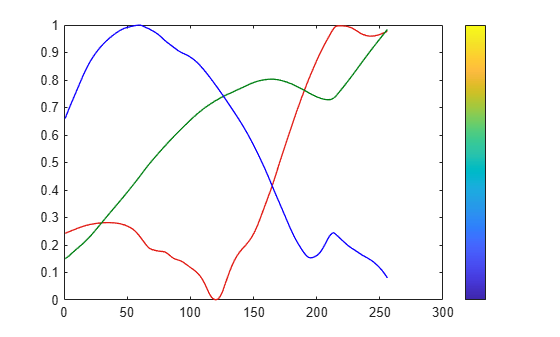rgbplot

Description

example

rgbplot(map) plots the red, green, and blue intensities of the specified colormap.

Examples

collapse all

Plot the parula colormap, and display a colorbar as a visual reference.

rgbplot(parula)
hold on
colormap(parula)
colorbar('Ticks',[])Create a custom colormap and plot it. Then display a colorbar as a visual reference.

r = (0:.1:.9)';
g = r.^1.8;
b = r.^2.1;
mymap = [r g b];
rgbplot(mymap)
hold on
colormap(mymap)
colorbar('Ticks',[])Input Arguments

collapse all

Colormap to plot, specified as a three-column matrix of RGB triplets. An RGB triplet is a three-element row vector whose elements specify the intensities of the red, green, and blue components of a color. The intensities must be in the range [0, 1]. For example, here is a colormap that contains five colors:

map = [0.2 0.1 0.5
0.1 0.5 0.8
0.2 0.7 0.6
0.8 0.7 0.3
0.9 1 0];

This table lists the RGB triplet values for common colors.

ColorRGB Triplet
yellow[1 1 0]
magenta[1 0 1]
cyan[0 1 1]
red[1 0 0]
green[0 1 0]
blue[0 0 1]
white[1 1 1]
black[0 0 0]

Alternatively, you can create the matrix by calling one of the predefined colormap functions. Call the function as the input argument to the rgbplot function. For example, this command plots the parula colormap.

rgbplot(parula)

Data Types: double | single